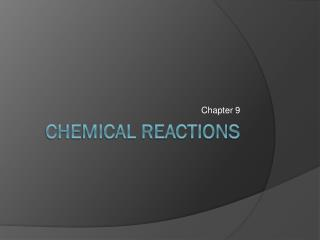DownloadDownload PresentationChemical Reactions

# Chemical Reactions

Télécharger la présentation## Chemical Reactions

- - - - - - - - - - - - - - - - - - - - - - - - - - - E N D - - - - - - - - - - - - - - - - - - - - - - - - - - -
##### Presentation Transcript

1. Chapter 9 Chemical Reactions

2. Section 1 Reactions & Equations

3. Chemical Reaction • The process by which the atoms of one or more substances are rearranged to form different substances.

4. How Can You Tell If A Chemical Reaction Has Taken Place? • Temperature change • Color change • Odor • Gas formation (Bubbles) • Formation of a solid

5. Representing Chemical Reactions • Chemists use statements called equations to represent chemical reactions. • These equations have two parts • Reactants – The starting materials • Products – The substances that are formed during a chemical reaction.

6. Word Equations • Example

7. Skeleton Equation • Example

8. Chemical Equations • A statement that uses chemical formulas to show the identities and relative amounts of the substances involved in a chemical reaction.

9. Balancing Chemical Equations • Recall that matter is neither created nor destroyed in a chemical reaction. • In order to follow the law of conservation of matter we must balance our chemical equations so that the reactant and product sides are equal to one another.

10. Coefficient – In a chemical equation is the number written in front of a reactant or product. • Example • Coefficients are usually whole numbers; the number 1 is usually omitted.

11. Steps for Balancing Equations • Write the skeleton for the reaction. • Count the atoms of the elements in the reactants. • Count the atoms of the elements in the product. • Change the coefficients to make the number of atoms of each element equal on both sides. • Write the coefficients in their lowest possible ratios. • Check your work!

12. Example: Balancing Chemical Equations

13. Section 2 Classifying Chemical Reactions

14. Types of Chemical Reactions • We will look at five types of chemical reactions. • Each type of reaction will have a general formula that will be helpful in recognizing the type of reaction present.

15. Synthesis Reaction • A chemical reaction in which two or more substances (A and B) react to produce a single product (AB). • General Form • Example

16. Combustion Reaction • In a combustion reaction oxygen combines with a substance and releases energy in the form of heat and light. • In a combustion reaction • is always present • Usual products are &

17. Decomposition Reaction • A reaction in which a single compound breaks down into two or more elements or new compounds. • General Form • Example

18. Single-Replacement Reaction • A reaction in which the atoms of one element replace the atoms of another element in a compound. • General Form • Example

19. Double-Replacement Reaction • A reaction in which ions between two compounds are exchanged. • General Form • Example

20. Helpful Hints… • Nonmetals replace nonmetals • Metals will replace Hydrogen or another metal. • More active metals will replace less active metals (see Figure 13 p.293). • A less active metal will not replace a more active metal.

21. Looking At Double-Replacement Reactions • Only occurs if one of the products is a solid precipitate. • Precipitate– an ionic compound formed by a double-replacement reaction • Which is not soluble in water • It forms a cloudy appearance in the solution; if allowed to sit, the precipitate will settle to the bottom of the container

22. Solubility Rules for Ionic Compounds • Always Soluble (aqueous)

23. Generally Insoluble (form precipitates)

24. Mostly Soluble (usually aqueous) • Use the Solubility Table on p.974

25. Reactions in Aqueous Solutions

26. As we have talked about previously a solution is a homogeneous mixture. • Many of the reactions discussed in the previous section involve substances dissolved in water. • When a substance is dissolved in water, a solution forms. • An aqueous solution contains one or more substances called solutes dissolved in water and water in this case would be the solvent.

27. What Happens In an Aqueous Solution? • Ions form • Example

28. Ionic Equations • To show the details of reactants that involve ions in aqueous solutions, chemists use ionic equations. • Ionic equations differ from chemical equations in that substances that are ions in solution are written as ions in the equation.

29. Complete Ionic Equation • An ionic equation that shows all of the particles in a solution as they exist. • Example

30. Spectator Ion • Ions that do not participate in a reaction. • Example

31. Net Ionic Equation • Ionic equations that include only the particles that participate in the reaction. • Spectator ions are not included • Example